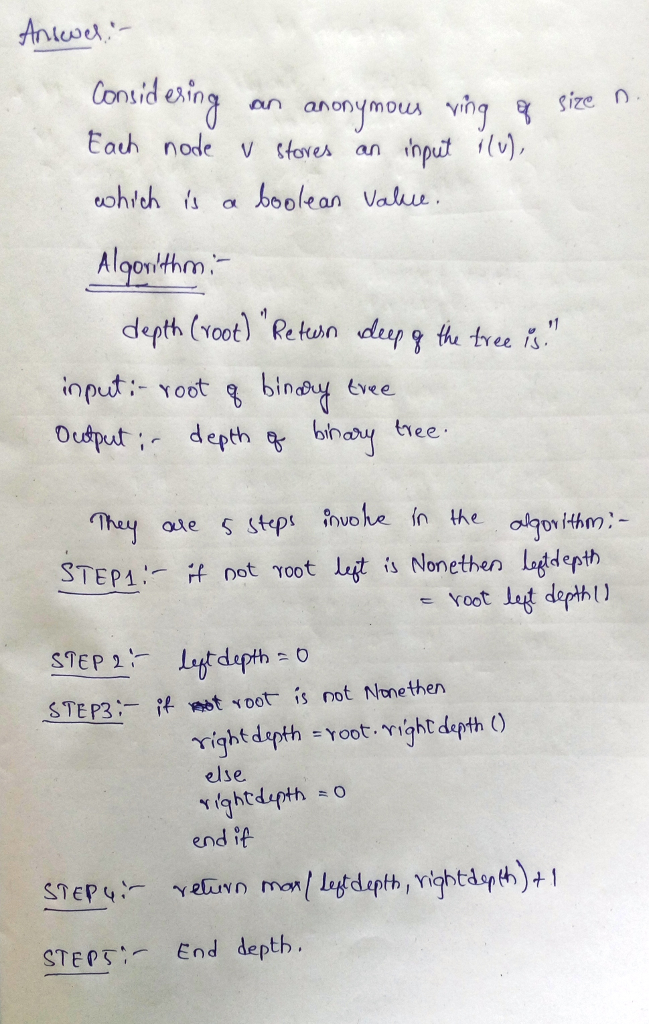# Homework Solution: Consider an anonymous ring of size n. Each node v stores an input i(v), which is a boolean value. When the algo…

Consider an anonymous ring of size n. Each node v stores an input i(v), which is a boolean value. When the algorithm terminates, then every node is to store the output in its private variable. Every node knows n, in that n can be a part of code. (a) Give a deterministic algorithm to compute the global boolean OR of all input values of the nodes of the ring. (b) Show that any such algorithm requires (n2) messages.